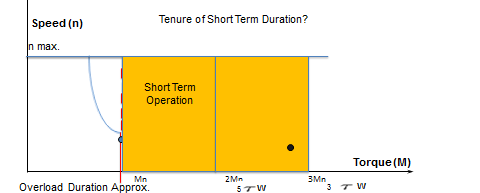Read: Part I  |  Part II

[continued...]

The thermal data given in our catalogue may be indicative, and it should always be confirmed whether the heat dissipation in a given application is actually better and stable. As per the speed-torque curve in Portescap’s catalogue, there is a temporary working range in which we can overload the motor for a small time span. However we should always confirm what that time span should be, and it all really depends on motor size and design. There is a definite time span for every motor size, and this limitation comes from the coil temperature and more precisely, how fast the motor coil or winding heats up.

The natural time scale is nothing but a thermal time constant of motor and winding. Practically, for smaller motors the time constant is in range of a few seconds, while for larger motors the same amounts to almost half of a minute. Let us see the below diagram where the torque is two times the maximum continuous torque. Here, the time constant for motor overload can be 20 to 25 seconds for our motor.  However, for motor overload of three times of maximum continuous torque, this time duration may be quite short.Factors affecting Thermal Time Constant of a DC Motor

We have already covered the limits of a motor in terms of maximum speed, maximum continuous torque & short term torque. Now we need to understand the importance of current in the application. Practically a motor heats up due to current & not torque. However there is a simple and directly proportional relation between current & torque given as:

M = kT x I mot

Torque constant kT has to be the proportionality factor for a given motor current and it’s torque equivalent. So T axis can also be replaced by I axis accordingly.

Current magnitude at maximum continuous torque is denoted by maximum continuous current in amperes. The torque constant is given in our catalogue page. Torque constant is the amount of torque in mNm produced when 1 ampere of current is passed.

We now understand that if we limit the current, the motor torque also can be limited. The power supplies and controllers used in a typical application may have some current limitations, which in turn result in limitations in associated torque. This would obviously affect the short term operating ranges and they might be restricted at that point. Since there is a limitation on current, the load operating points cannot be smoothly handled by the motor which they are designed for.

In summary,

• Every type & size of motor has a speed limit which depends mainly upon the life of brush & bearings.
• Motor torque & current are in directly proportional, and the relation between them is given by the torque constant.
• Motors have torque limits, i.e. there is a continuous limit of torque denoted by maximum continuous torque or current.
• Motors also have a temporary working range or short term operation which is limited by time. The time that limits the short term operations is known as the thermal time constant.